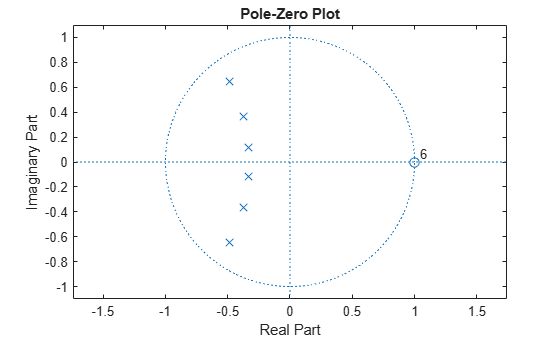isstable

Determine whether filter is stable

Syntax

flag = isstable(b,a)
flag = isstable(sos)
flag = isstable(d)

Description

flag = isstable(b,a) returns a logical output, flag, equal to true if the filter specified by numerator coefficients, b, and denominator coefficients, a, is a stable filter. If the poles lie on or outside the circle, isstable returns false. If the poles are inside the circle, isstable returns true.

flag = isstable(sos) returns true if the filter specified by second order sections matrix, sos, is stable. sos is a K-by-6 matrix, where the number of sections, K, must be greater than or equal to 2. Each row of sos corresponds to the coefficients of a second order (biquad) filter. The ith row of the sos matrix corresponds to [bi(1) bi(2) bi(3) ai(1) ai(2) ai(3)].

flag = isstable(d) returns true if the digital filter, d, is stable. Use designfilt to generate d based on frequency-response specifications.

Examples

collapse all

Design a sixth-order Butterworth highpass IIR filter using second order sections. Specify a normalized 3-dB frequency of 0.7. Determine if the filter is stable.

[z,p,k] = butter(6,0.7,'high');
SOS = zp2sos(z,p,k);
flag = isstable(SOS)
flag = logical
1

zplane(z,p)Redesign the filter using designfilt and check it for stability.

d = designfilt('highpassiir','DesignMethod','butter','FilterOrder',6, ...
'HalfPowerFrequency',0.7);
dflg = isstable(d)
dflg = logical
1

zplane(d)Create a filter and determine its stability at double and single precision.

b = [1 -0.5];
a = [1 -0.999999999];
act_flag1 = isstable(b,a)
act_flag1 = logical
1

act_flag2 = isstable(single(b),single(a))
act_flag2 = logical
0